Courses

# Newton's Law Of Motion MCQ Level - 1

## 10 Questions MCQ Test Topic wise Tests for IIT JAM Physics | Newton's Law Of Motion MCQ Level - 1

Description
This mock test of Newton's Law Of Motion MCQ Level - 1 for IIT JAM helps you for every IIT JAM entrance exam. This contains 10 Multiple Choice Questions for IIT JAM Newton's Law Of Motion MCQ Level - 1 (mcq) to study with solutions a complete question bank. The solved questions answers in this Newton's Law Of Motion MCQ Level - 1 quiz give you a good mix of easy questions and tough questions. IIT JAM students definitely take this Newton's Law Of Motion MCQ Level - 1 exercise for a better result in the exam. You can find other Newton's Law Of Motion MCQ Level - 1 extra questions, long questions & short questions for IIT JAM on EduRev as well by searching above.
QUESTION: 1

### A bob is hanging over a pulley inside a car through a string. The second end of the string is in the hand of a person standing in the car. The car is moving with constant acceleration a directed horizontally as shown in figure. Other end of the string is pulled with constant acceleration a vertically downward. The tension in the string is equal to :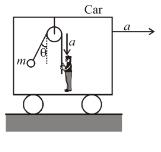Solution: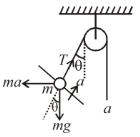Free Body diagram in the frame of the car
Applying Newton’s law perpendicular to string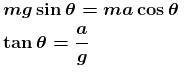Applying Newton’s law along string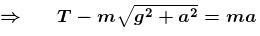The correct answer is: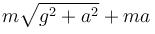QUESTION: 2

### A man is standing on a spring balance platform. Reading of balance is 60 kg wt. If the man jumps outside the platform, then the reading of the balance :

Solution:

The man will first press the platform to get extra normal force required for the jump. Hence initially the reading will be greater than 60 kg wt and then decrease to zero.

The correct answer is: Becomes > 60 kg-wt initially and then decreases to zero

QUESTION: 3

### The elevator shown in figure is descending, with an acceleration of 2m/s2. The mass of block A is 0.5 kg. The force exerted by block A on block B is (mass of block B is 1 kg)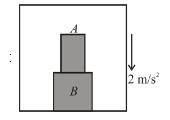Solution: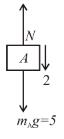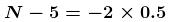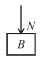N = 4N

QUESTION: 4

In the figure shown, a person wants to raise a block lying on the ground to a height h. In both the cases if time required is same then in which case he has to exert more force. Assume pulleys and strings light.(let F1 be force in (i) and F2 be force in (ii))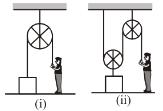Solution: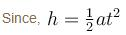⇒  a should be same in both case, because h and t are same in both cases as given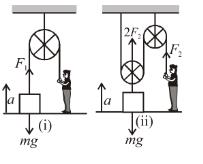In figure (i)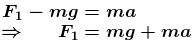In figure (ii)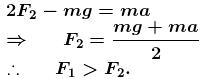QUESTION: 5

A spring of negligible mass going over a clamped pulley of mass  m  supports a block of mass M as shown in the figure. The force on the pulley by the clamp at the time of equilibrium is given by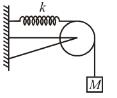Solution:

Free body diagram of pulley is shown in figure. Pulley is equilibrium under four forces. Three forces as shown in figure and the fourth, which is equal and opposite to the resultant of these three forces, in the force applied by the clamp on the pulley (say F).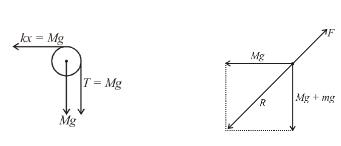From the F.B.D we obtain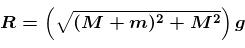Therefore, the force F is equal and opposite of R as shown in figure.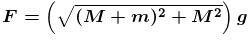The correct answer is: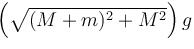QUESTION: 6

A uniform rope of length L and mass M is placed on a smooth fixed wedge as shown. Both ends of rope are at same horizontal level. The rope is initially released from rest, then the magnitude of initial acceleration of rope is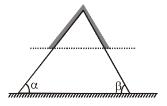Solution: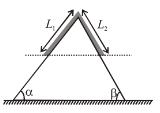Let L1 and L2  be the portions (of length) of rope on left and right surface of wedge as shown
∴  magnitude of acceleration of rope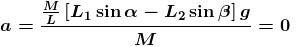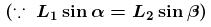QUESTION: 7

Two blocks A and B of masses  m  and  2m  respectively are held at rest such that the spring is in natural length. Find out the accelerations of blocks A and B respectively just after release (pulley, string and the spring are massless).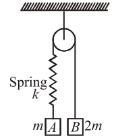Solution:

Let us assume that the block A of mass m is tied to the spring. However at t=0s, the spring is not compressed or extended.

Let T be the tension in the string going over pulley.   Just after releasing the force on block A, both blocks move with the same acceleration. The tension is same in the rope on both sides.  The equations of motion for both blocks are:

T−mg=ma

2mg−T=2ma

So  mg=3ma=>a=g/3

So at t = 0, block A moves up initially with an acceleration g/3 and block B moves down with acceleration g/3.

QUESTION: 8

Two particles of mass m  each are tied at the ends of a light string of length 2a. The whole system is kept on a frictionless horizontal surface with the string held light so that each mass is at a distance a from the centre P (as shown in the figure). Now, the mid point of the string is pulled vertically upwards with a small but constant force F. As a result, the particles move towards each other on the surface. The magnitude of acceleration, when the separation between them becomes 2x, is :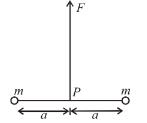Solution: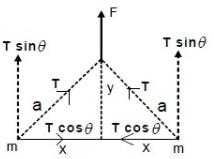As shown in the FBD resolving the forces along the vertical and horizontal axis in the final position we have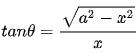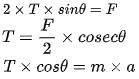Putting in the values and solving we get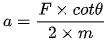Putting in the value of cotθ as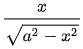we have the option C as the correct one.

QUESTION: 9

A person is standing in an elevator. The situation in which he finds his weight less than actual is?

Solution:

Reason: Newton's third law of motion.  If a body exerts a force on another, there is an equal and opposite force called a reaction on the first body by the second. In Downward moving elevator (with a uniform acceleration), the man experiences an upward force. This reduces his weight.
In case of uniform velocity, Force= mas x acceleration is zero since acceleration is zero.

QUESTION: 10

Block of mass  M1 and M2  are connected by a cord which passes over the pulleys P1 and P2 as shown in the figure. If there is no friction, the acceleration of the block of mass M2 will be :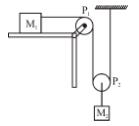Solution: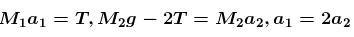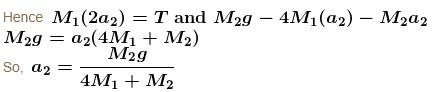The correct answer is: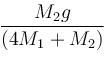Track your progress, build streaks, highlight & save important lessons and more!

### Similar Content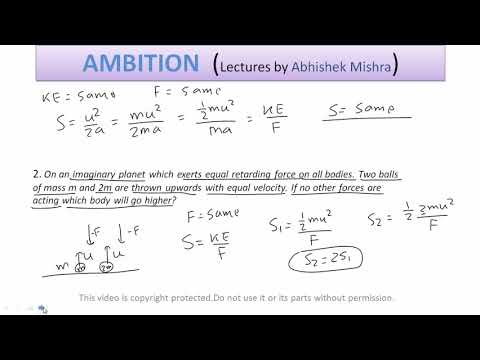### Related tests